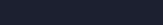# MySQL true false type column

MySQL does not have a boolean column type, there is no way to systematically store true/false in a database. Except tinyint comes and saves the day. With tinyint using 1 to represent true and 0 to represent false is the unofficial way of storing booleans.

It makes sense, 1 means yes/go/open/set whilst 0 has the meaning of no/stop/closed/unchecked.

Naturally, 0 is equal to false and 1 is true, however many other variables and objects can be false like an empty string, 0.0 float or an array with no elements, whilst true can be accepted from mostly everything else.

Assume this MySQL table:

```\$uid = 1323;
\$select = \$db->prepare("SELECT `verified` FROM `status` WHERE `uid` = :uid LIMIT 1;");
\$select->execute(array(':uid' => \$uid));
\$row = \$select->fetch();```

and then checking if true or false with an if statement:

```if (\$row['verified']){
echo "Verified";
} else {
echo "Not verified";
}```

As verified is 0 for uid 1323 running the code gives “Not verified”.  0 means false whilst 1 would be true.

Updating the uid 1323 to be verified could just be done by setting it to = 1 however updating it as true has the same effect:

```\$update = \$db->prepare("UPDATE `status` SET `verified` = :verified WHERE `uid` = :uid LIMIT 1;");
\$update->execute(array(':verified' => true, ':uid' => \$uid));```

Verified = 1 for uid 1323.

The same goes for an insert query:

```\$insert = \$db->prepare("INSERT INTO `status` (`uid`, `verified`) VALUES (?, ?)");
\$insert->execute([1326, true]);```

Creating a new entry for uid 1326 and setting verified as true:In a sense, the only thing that can throw you of is the lack of visual clue being TRUE/FALSE however as you have seen 1/0 is the same thing, it takes up less space too.